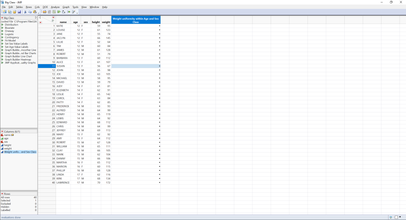Choose Language Hide Translation Bar
Highlighted

## Formula within a same categories of data

Hi,

Im new to JSL and JMP, and i have a problem to write a formula to process a raw data that i have:

For example for the Big Class Sample data, I would like to create a formula of "Weight uniformity within a the same age and sex ".

The formula would be

Weight Uniformity = [Weight - (average weight for same age and sex)] / Weighthope that some expert members could help me on this.

thanks

Regards

Ashraff

1 ACCEPTED SOLUTION

Accepted Solutions
Highlighted

## Re: Formula within a same categories of data

Here is the formula

``(:weight - Col Mean( :weight, :age, :sex )) / :weight``

Documentation for the Col Mean() function can be found in the Scripting Index

Help=>Scripting Index

Jim
2 REPLIES 2
Highlighted

## Re: Formula within a same categories of data

Here is the formula

``(:weight - Col Mean( :weight, :age, :sex )) / :weight``

Documentation for the Col Mean() function can be found in the Scripting Index

Help=>Scripting Index

Jim
Highlighted

## Re: Formula within a same categories of data

Hi Jim,

Thanks. That worked beautifully!
Article Labels

There are no labels assigned to this post.